﻿ New Methodology for the Prediction of Motor Starting Effect on Bus Voltages of Interconnected Power Systems

### New Methodology for the Prediction of Motor Starting Effect on Bus Voltages of Interconnected Power ...

Alexis Polycarpou, Hassan Nouri, Ali Azizpour

American Journal of Electrical and Electronic Engineering

## New Methodology for the Prediction of Motor Starting Effect on Bus Voltages of Interconnected Power Systems

Alexis Polycarpou1, Hassan Nouri2,, Ali Azizpour3

1Department of Electrical Engineering, Frederick University Cyprus, Palouriotissa, 1036, Nicosia, Cyprus

2Power Systems, Electronics and Control Research Laboratory, UWE Bristol BS16 1QY, UK

3Faculty of Electrical and Computer Engineering, University of science and technology of Mazandaran, Behshahr, Iran

### Abstract

A new methodology is proposed in this paper capable of predicting the impact of induction motor load starting on the bus voltages of an interconnected power system. The profile of the voltage sag is predicted for each bus, which is used to improve the power quality of a system. The methodology is investigated with the use of a four bus, as well as fourteen bus IEEE interconnected system. Mathematical and simulation results demonstrate the effectiveness and applicability of the proposed methodology for the application.

• Alexis Polycarpou, Hassan Nouri, Ali Azizpour. New Methodology for the Prediction of Motor Starting Effect on Bus Voltages of Interconnected Power Systems. American Journal of Electrical and Electronic Engineering. Vol. 4, No. 5, 2016, pp 139-147. http://pubs.sciepub.com/ajeee/4/5/3
• Polycarpou, Alexis, Hassan Nouri, and Ali Azizpour. "New Methodology for the Prediction of Motor Starting Effect on Bus Voltages of Interconnected Power Systems." American Journal of Electrical and Electronic Engineering 4.5 (2016): 139-147.
• Polycarpou, A. , Nouri, H. , & Azizpour, A. (2016). New Methodology for the Prediction of Motor Starting Effect on Bus Voltages of Interconnected Power Systems. American Journal of Electrical and Electronic Engineering, 4(5), 139-147.
• Polycarpou, Alexis, Hassan Nouri, and Ali Azizpour. "New Methodology for the Prediction of Motor Starting Effect on Bus Voltages of Interconnected Power Systems." American Journal of Electrical and Electronic Engineering 4, no. 5 (2016): 139-147.

 Import into BibTeX Import into EndNote Import into RefMan Import into RefWorks

12345
Prev Next

### 1. Introduction

Voltage sag is defined as an rms variation with a magnitude between 10 % and 90 % of nominal and a duration between 0.5 cycles and one minute , and is considered to be one of the most serious power quality issues. Voltage sags are caused by a increase in transient current, usually appearing during motor starting, transformer energizing, load switching and faults. The probability of equipment failure due to voltage sag and power system quality variations in general increases dramatically with increased sensitivity. As technology evolves, equipment such as adjustable speed drives, process control equipment and computers are designed with the use of highly sensitive modern power electronics and inevitably become more vulnerable.

The influence of induction motor loads on the characterization of voltage sags is of great importance, as indicated by researchers [2-10]. Motors decelerate during a short circuit, and for a time interval behave as generators, maintaining a voltage supplied by its internal or back electromotive force (e.m.f). After fault clearance, the motor will accelerate again, drawing a high reactive current from the supply, until the steady-state speed is reached, causing prolonged post fault voltage sag. The deceleration and acceleration of motors influences the duration and shape of voltage sags. Most studies characterizing and predicting voltage sags are based on statistical data describing the frequency of occurrence, the magnitude and duration of the sags [11, 12, 13, 14]. Recent studies have produced voltage sag indices mathematically describing the sag and providing tools for online calculation techniques [15, 16, 17]. Those indices however are applicable on radial systems. Once the power system under investigation becomes interconnected, the application of those indices is no longer easily performed. Thus the need of a methodology, which can be used to predict voltage sags caused by large load switching and motor starting at interconnected systems, arises.

### 2. Methodology of Analysis

The purpose of the methodology presented is to predict the depth, duration, and the profile of a voltage sag sensed on the buses of an interconnected power system due to large induction motor load switching on one of the system buses. The large induction motor load used in the investigation is developed and verified in . The proposed methodology consists of three steps :

• Step 1 - Calculation of ZPCC (Impedance at point of common coupling).

• Step 2 - Obtaining power waveforms.

• Step 3 - Instantaneous load flow calculation.

The methodology proposed is shown on a flowchart depicted in Figure 1, and theoretical considerations regarding each step are discussed in the following sections.

2.1. Step 1- Calculation of ZPCC

The power system transmission lines data are used in order to calculate the impedance at the point of common coupling (ZPCC) in this step. Each bus of the system has its own characteristic ZPCC.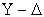and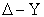transformation can be used to reduce the impedances between the equivalent generator bus and the load bus, identifying the value of ZPCC. Figure 2 and equation (1) illustrates the principle ofandtransformation.Download asVeiw figureFigures index
Figure 1. Flow chart representation of the methodology used in producing the transient voltage response on the system busesDownload asVeiw figureFigures index
Figure 2.andtransformation

The equations used in the transformations, referring to Figure 1 are shown in equations (1) - (2).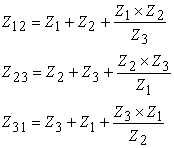(1)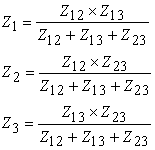(2)
2.2. Step 2- Obtaining Power Waveforms

The ZPCC value calculated in the previous section can be used to represent the impedance between the generator and the bus which the load is being supplied by, as illustrated in Figure 3.Download asVeiw figureFigures index
Figure 3. Equivalent circuit for power calculation

The test system shown in Figure 3 is used in order to identify the active and reactive power demand of the motor load. Equations (3) and (4) express the rate of change of a variable at time ‘t’ as a function of the value of the same variable at time ‘t-1’. They describe the motor transient response in terms of direct and quadrature axis currents and voltages [12, 13]. The parameters in the equations correspond to Figure 4. The motor load will be switched in an already energized system. The current flow and bus voltages before switching are known, as they are the steady state load flow solutions of the system. These voltages and currents are used in the first time step solution of equations (3)-(6) as the variables corresponding to time ‘t-1’. Thus the value of Id and Iq parameters for time ’t’ can be calculated (This is explained in Appendix A: Basic Machine Theory).Download asVeiw figureFigures index
Figure 4. Induction motor d-q axis quantities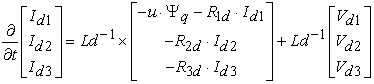(3)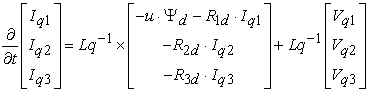(4)

Using these values and Park’s reference frame transformation equations shown in equations (5) and (6), the values of the three phase voltages and currents can be obtained.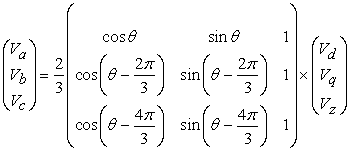(5)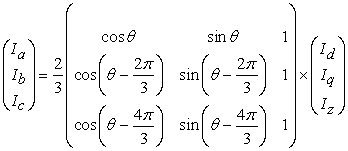(6)

The values of active (P) and reactive power (Q) can be calculated for the particular time step using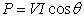and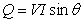, respectively. For the calculation of the next time step, ‘t+1’, the value of current at time ‘t’ has already been found in the previous step. As the per unit voltage at the motor terminals will be the one sent minus the voltage drop along ZPCC, the voltage value at time step ‘t’ can be calculated using.

The process defined above is repeated until the voltage and current variables vary in a rate less than the accepted error margin for the specific application and thus have reached steady state. Having obtained the active and reactive power demand of the motor, the values of active and reactive power, as well as transmission line impedance can be used to define the increment of power generated within the whole system. The increment of power can be allocated to the generators according to their MVA and their relative electrical distance from the load bus under consideration. The increment in power is then added to the steady state power generation pre-switching, which is known. Thus the active and reactive power transient waveforms of the induction motor and the system generators can be obtained, and are used in step three of the proposed methodology. The assumption made for the calculation of the active and reactive power waveforms is that the generators and the system do not enter an instability region during the transient period of switching, and the moment of inertia of all machines is the same. A more detailed model of the generators can be used to identify the generator response of a system with different parameters.

2.3. Step 3- Instantaneous Load Flow Calculation

Load flow is a methodology which provides a solution to a radial or meshed power system. There are various methods through which load flow solutions can be obtained. Typical iterative methods are Gauss-Seidel, Newton-Raphson, and Decoupled load flow method.

The load flow technique used in this step can be any of the above depending on the level of accuracy and speed required by the user for the application. It was decided for illustration reasons to use the Gauss-Seidel method as it has been widely used and it is easily applicable for a wide range of systems. In the Gauss-Seidel method, the system bus voltages are assumed at the first iteration, and the values of those quantities obtained during calculation are used in the second iteration. For this method at least one of the generator buses of the system is assumed to be an infinite bus with constant voltage and frequency. A generalize expression representing this method is shown in (7). The procedure of iterations is generally repeated until an acceptable error margin of variance appears for all unknown variables between two iterations.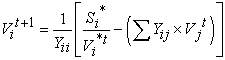(7)

where V is the voltage, t is the iteration step, i is the bus under consideration, j is the other buses in the system, Y is the admittance of transmission lines, and S is the bus apparent power.

The proposed methodology introduces the concept of inserting the transient response active and reactive power values obtained during Step 2, as discrete time step inputs in the load flow technique to produce the transient voltage response on the system buses. In large systems, the amount of data produced can be time consuming and also complicated. Thus a time step can be defined by the sensitivity of the customers connected to the system buses. A large increment of time step could cause a reduction in the accuracy of the resulting waveforms. Thus the depth and duration of the voltage sag inflicted by the motor switching on all buses can be obtained from the output of the series of load flow solutions.

In the next sections of this paper, an attempt is made to illustrate the validity and accuracy of the proposed method on interconnected systems using PSCAD  and Mathcad.

### 3. Methodology Application

The methodology proposed in the previous section is applied and verified with the use of two interconnected power system test cases. The first is a four bus interconnected power system, and the second is the IEEE 14 bus interconnected test system. The validation procedure and results obtained for the systems are shown in the following sections.

3.1. Four Bus Interconnected Power System

The four bus interconnected power system is shown in Figure 5. Buses 0 and 1 have generators connected to them, whereas buses 2 and 3 are supplying loads. The voltage sag is caused in the system by the introduction of a new load on bus 2, (Zl) consisting of induction motors. The four-bus system parameter datasheet is available upon request.Download asVeiw figureFigures index
Figure 5. The Four bus interconnected system, with induction motor load on bus 2.

3.1.1. Step 1-Calculation of ZPCC

In order to calculate ZPCC, the power system impedances are reduced to an equivalent impedance between the load bus causing the sag (in this case bus 2) and the generator equivalent bus, as described in the previous section. The calculations performed to identify ZPCC were carried out in MathCAD. The ZPCC value of the load bus 2 is: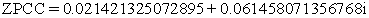(8)

where the letter ‘i’ in MathCAD represents the imaginary notation ‘j’.

3.1.2. Step 2- Obtaining Power Waveforms

In this section, the active and reactive power demand waveforms are obtained for the starting period of the induction motor individual generators’ contribution also calculated. The value of ZPCC obtained in the previous section can be incorporated into a two-bus equivalent circuit as shown in Figure 3. The motor active and reactive power demand waveforms are obtained using equations (3)-(6) as described in a previous section. The calculated active and reactive motor load power demand with respect to time is shown in Figure 6. The active and reactive power waveforms shown in Figure 6 are causing the voltage sag, as they will be demanded on the load bus. Thus generators will have to increase their production to fulfill that demand.

The numerical value of the current, as well as active and reactive power load waveforms are used to produce the instantaneous values of the generator at specific time steps. The time step is decided according to the desired accuracy of the user. In the case where speed of calculation is preferred over accuracy the time step of the waveform used in the method can be increased. Table 1 illustrates the active and reactive power results calculated for G2, with G1assumed to be infinite bus.Download asVeiw figureFigures index
Figure 6. Calculated motor load power demand.

#### Table 1. Calculated G2 Power Values

3.1.3. Step 3- Instantaneous Load Flow Calculation

Having established the active and reactive power numerical values for the motor and the system generators, the data are incorporated in MathCAD, where a mathematical program calculates the values of the resulting voltages for each iteration of Gauss-Seidel. When the error between the voltages of two consecutive iterations is less than the user pre-defined value, then the convergence criterions are fulfilled and a solution is reached. The load flow MathCAD program is shown in Figure 7. It can be seen from Figure 7 that the error of accuracy used while obtaining the results was 0.00001 %. In the case where speed of calculation is preferred over accuracy, the time step of the waveform used in the program can be increased and the error acceptability margin decreased.Download asVeiw figureFigures index

For each time step of the input active and reactive power waveforms, a number of iterations are performed until the solution is reached. The next point on the waveform is then inserted into the program as a numerical value and the process repeats itself until the complete waveform is plotted. The obtained results for the case studied in this section in terms of voltage level on the load buses during the voltage sag is presented in Table 2.

3.1.4. Step 4- Four Bus Results Verification

In order to verify the proposed methodology, the four-bus system was simulated in PSCAD. The values of the bus voltages were recorded for the duration of the sag. A comparison of the results using the proposed method and the simulation is shown in Figure 8.

Observing the results shown in Figure 8, it can be concluded that the results of the mathematical methodology proposed are very close to the simulated results. The maximum deviation of results is measured to be 0.7 %. Thus it can be concluded that the methodology is accurate when applied to this test system. Next, the methodology proposed is applied on a larger system, such as the fourteen bus IEEE system.Download asVeiw figureFigures index
Figure 8. Comparison of results from proposed methodology and PSCAD simulation
3.2. Fourteen Bus IEEE Test System

The methodology proposed is applied on the IEEE fourteen bus interconnected system in an attempt to further validate its accuracy. The aim is to further validate its accuracy of the proposed methodology. The validation procedure and results are shown in the next sections. The fourteen-bus IEEE interconnected system is presented in Figure 9. The system parameter values are available upon request .

As shown in Figure 9, the test system consists of fourteen buses, with buses 1and 2 having generators connected to them, and buses 3, 6 and 8 being supplied by synchronous condensers. The voltage sag is caused in the system by the introduction of a new load ‘Zl’ on bus fourteen of the system, consisting of induction motors . The steps of the proposed methodology are applied to the system in order to illustrate its applicability and accuracy.Download asVeiw figureFigures index
Figure 9. The IEEE fourteen-bus interconnected system

3.2.1. Step 1- Calculation of ZPCC

In order to calculate the ZPCC, the power system impedances are reduced to one equivalent impedance between the load bus causing the sag (in this case bus 14), and the generator equivalent bus, as described previously. The fourteen-bus system shown in Figure 9 for a load supply point on bus 14 can be reduced in a similar way as previously for the four bus test system. The resulting per unit value of ZPCC for this case is calculated to be:

Having obtained the value of ZPCC, Step 2 of the proposed methodology is performed.

3.2.2. Step 2- Obtaining Power Waveforms

In this section, the active and reactive power demand waveforms are obtained, for the transient period of the induction motor. The contribution of the generators towards that power demand is also calculated. The value of ZPCC, obtained in the previous section, can be incorporated in a two bus equivalent circuit as shown in Figure 2. The motor demand active and reactive power waveforms can be obtained in the same way as for the four bus system, and are shown in Figure 10.Download asVeiw figureFigures index
Figure 10. Motor load power demand for load switching on bus 14

The generators of the system have to increase the power generated to incorporate the increased demand caused by the switching in of the motor. It is necessary for the application of the methodology to identify the contribution of each of the generators towards the demand increase. The numerical values of the active and reactive power load waveforms were used to produce the value of the generator power waveforms as in the previous case. Having calculated the values of load and generator power, and the value of current according to equations (2)-(5), the generator active and reactive power calculated are shown in Figure 11 and Figure 12, respectively. The transient period is assumed to start at 10 seconds as the system under study needs to initialize first.Download asVeiw figureFigures index
Figure 11. System generators’ active power (MW) with load switching on bus 14Download asVeiw figureFigures index
Figure 12. System generators reactive power (MVAR) with load switching on bus 14

3.2.3. Step 3- Instantaneous Load Flow Calculation

Having established the active and reactive power numerical values for the motor and the system generators, the transmission line and load data were used in a Mathcad program. The program implementing Gauss-Seidel technique in Mathcad is an extension of the program shown in Figure 7.

The obtained results from the program for the case studied in this section are presented in Table 3, for buses 2-7 and Table 4, for buses 8-14. In the above tables, the time in seconds and the voltage on the load buses in per unit values are shown during the voltage sag.

#### Table 4. Methodology Results for IEEE Test System (Buses 8-14)

3.2.4. Step 4- Instantaneous Load Flow Calculation

In order to verify the proposed methodology and the result obtained through the load flow technique shown in Table 3 and Table 4, the fourteen-bus system was simulated in PSCAD, with the motor load switched in the system at bus fourteen. The bus voltage values were recorder for the time steps used in the proposed method. A comparison of the calculated results using the proposed method and the obtained simulation results is shown in Figure 13. The comparison results are shown in terms of maximum accuracy deviation for each bus. The data is presented in this form due to the large amount of data produced. Detailed data are available upon request. The results in Figure 13 show that the maximum error between calculation and simulation is less than 1.2 %. Therefore it is once again verified that the methodology proposed is within acceptable limits and can be used in predicting voltage sag occurring on the system buses, caused by heavy load switching. Thus it can be concluded that the methodology is accurate when applied to this test system.Download asVeiw figureFigures index
Figure 13. Maximum percentage error between proposed methodology and PSCAD simulation for connecting the load on bus 14

### 4. Conclusions

The various steps of the proposed methodology have been discussed, explained, and validated using a four bus system and the IEEE fourteen bus interconnected test systems. For validation purposes, the proposed methodology was compared and validated through PSCAD simulation and MathCAD mathematical programming. It is shown that the calculated output of the methodology accurately represents the voltage sag sensed on the various buses. Further work would be accommodate the solution of all the mathematical expressions of the methodology proposed into one common environment to provide a complete package for voltage sag prediction caused by large induction motors for interconnected systems.

### Appendix A: Basic Machine Theory

The generalized machine model transforms the stator windings into equivalent commutator windings, using the dq0 transformation as follows: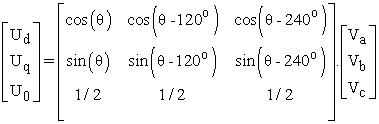The three-phase rotor winding may also be transformed into a two phase equivalent winding, with additional windings added to each axis to fully represent that particular machine, as is shown in Figure 4 [20, 21].

Where,

k = Amortisseur windings

f = Field windings

abc = Stator windings

d = Direct-Axis (d-axis) windings

The d-axis equivalent circuit for the generalized machine is shown in figures below. The second figure illustrates the flux paths associated with various d-axis inductances: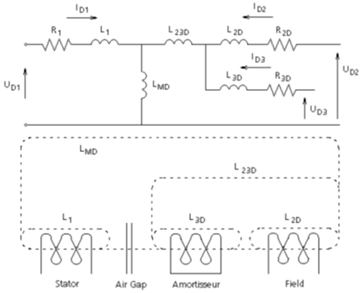Referring to the above figures, the following equations can be derived: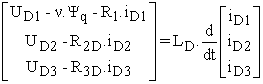Where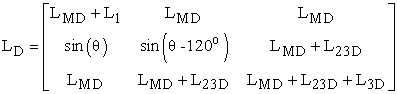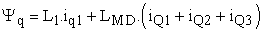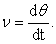Similar equations hold for the q-axis except the speed voltage term ν. Ψd, is positive, and: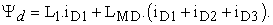Inversion of the first Equation gives the standard state variable form X0 = AX + BU with state vector X consisting of the currents, and the input vector U, applied voltages. That is: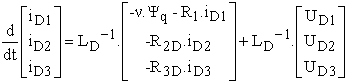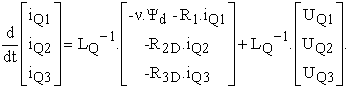The above equations are easy to integrate and hence are solved using trapezoidal integration to obtain the currents. The torque equation is given as: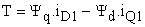and the mechanical dynamic equation for motor operation is: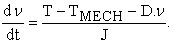### References

  IEEE, “Recommended practice for monitoring electric power quality”, IEEE, pp. 1-80, 1995.In article  Polycarpou, A, Nouri, H, Davies, T and Ciric, R “An overview of voltage sag theory, Effects and Equipment compatibility”, UPEC, Bristol, UK, 2004.In article  JMilanovic, J.V, Vegunta,S.C, Aung, M. T “The Influence of Induction Motors on Voltage Sag Propagation-Part I: Accounting for the Change in Sag Characteristics”, IEEE Transactions on Power Delivery, vol. 23, No. 2, pp. 1063-1071, 2008.In article View Article  JMilanovic, J.V, Vegunta,S.C, Aung, M. T “The Influence of Induction Motors on Voltage Sag Propagation-Part II: Accounting for the Change in Sag Performance at LV Buses”, IEEE Transactions on Power Delivery, vol. 23, No. 2, pp. 1072-1078, 2008.In article View Article  Huweg, A. F, Bashi, S. M.M and Mariun, S.N “Application of inverter based shunt device for voltage sag mitigation due to starting of an induction motor load”, CIRED 2005, 18th International Conference and Exhibition on Electricity Distribution, pp. 1-5, 2005.In article  Hsu, C.T, Chuang, H.J and Chen, C.S “Power Quality Assessment of Large Motor Starting and Loading for the Integrated Steel-Making Cogeneration Facility”, Industry, IEEE Transactions on Applications, vol. 43, No. 2, pp. 395-402, 2007.In article View Article  Polycarpou, A and Nouri, H “Analysis and simulation of bus loading conditions on voltage sag in an interconnected network”, UPEC, Staffordshire University, UK, 2002.In article  Bollen, M.H.J “The Influence of Motor Reacceleration on Voltage Sags”, IEEE Transactions on Industry Applications, vol. 31, pp. 667-674, 1995.In article View Article  Gomez, J.C, Morcos, M.M, Reineri, C and Campetelli, G, “Induction Motor Behavior Under Short Interruptions and Voltage Sags”, IEEE Power Engineering Review, pp. 11-15, 2001.In article View Article  Nouri, H and Polycarpou, A “The influence of double cage motors on voltage sag and power quality”, Medpower, Athens , Greece, 2002.In article PubMed  Becker, C et al., “Proposed Chapter 9 for Predicting Voltage Sags (Dips) in Revision to IEEE Std 493, the Gold Book”, IEEE Transactions on Industry Applications, vol. 30, pp. 805-821, 1994.In article View Article  Bollen, M.H.J. “Voltage Sags in Three-Phase Systems”, IEEE Power Engineering Review, pp. 8-15, September 2001.In article View Article  Yalcinkaya, G, Bollen, M.H.J and Crossley, P.A. “Characterization of Voltage Sags in Industrial Distribution Systems”, IEEE Transactions on Industry Applications, vol. 34, pp. 682-688, 1998.In article View Article  Bollen, M.H.J. “Voltage sag indices-Draft 2”, working document for IEEE P1564, November 2001.In article  Nouri, H and Polycarpou, A “Mathematical development, Investigation and Simulation of a new Quadratic Voltage Index”, UPEC, Newcastle, September 2006.In article  Polycarpou, A and Nouri, H “Investigation into the compatibility and effectiveness of a new mathematical online voltage sag index”, Int. Journal Power and Energy Conversion, vol. 2, No. 1, pp. 46-58, 2010.In article View Article  Polycarpou, A and Nouri, H “A New Index for On Line Critical Voltage Calculation of Heavily Loaded Feeders”, PowerTech, St. Petersburg, Russia, 2005.In article  Polycarpou, A and Nouri, H “Validation of a Proposed Voltage Sag Prediction Methodology for Interconnected systems during Motor Starting”, IEEE 44th Int. Universities Power Engineering Conference, Glasgow, Scotland, UK, September 2009.In article  Polycarpou, A “Identification of Voltage Sag Indices in Electrical Power Systems”, PhD Thesis, UWE, UK, April 2006.In article  PSCAD USER’S GUIDE, “A Comprehensive Resource for EMTDC” 211 Commerce Drive, Chapter 7: Rotating Machines, pp 126-132.In article  I. M. Canay, “Causes of Discrepancies on Calculation of Rotor Quantities and Exact Equivalent Diagrams of the Synchronous Machine,” IEEE Transactions, Vol. PAS-88, No.F, p. 1114-1120, July 1969.In article